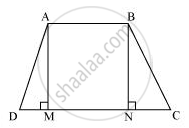# If the Non-parallel Sides of a Trapezium Are Equal, Prove that It is Cyclic. - Mathematics

If the non-parallel sides of a trapezium are equal, prove that it is cyclic.

#### SolutionConsider a trapezium ABCD with AB | |CD and BC = AD.

Draw AM ⊥ CD and BN ⊥ CD.

In ΔAMD and ΔBNC,

∠AMD = ∠BNC (By construction, each is 90°)

AM = BN (Perpendicular distance between two parallel lines is same)

∴ ΔAMD ≅ ΔBNC (RHS congruence rule)

∴ ∠ADC = ∠BCD (CPCT) ... (1)

∠BAD + ∠BCD = 180° [Using equation (1)]

This equation shows that the opposite angles are supplementary.

Therefore, ABCD is a cyclic quadrilateral.

Is there an error in this question or solution?
Chapter 10: Circles - Exercise 10.5 [Page 185]

#### APPEARS IN

NCERT Class 9 Maths
Chapter 10 Circles
Exercise 10.5 | Q 8 | Page 185

Share